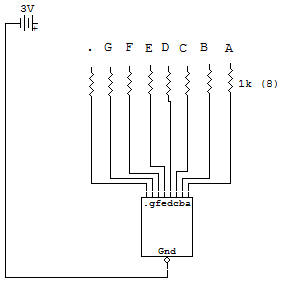Seven-Segment (LED) Digital Display Circuit (7 Seg)This is the beginning of the section on LED Digital Display Circuits see Diode Section.

You'll perform some very basic circuit experiments with the LED Display and we hope you learn how to understand and use the exciting component.

The purpose of this project is to learn the basic operation of a common-cathode, seven-segment, LED Digital Display. The function of a readout device is to change electrical signals into a visual display.

The simplest of readouts is a pilot light to tell us when power is ON in a circuit. The seven-segment readout was made in order to display the numerals 0,1, 2, 3, 4, 5, 6, 7, 8 and 9 for reading the output of a computer or calculator.

Seven segments (or separate lines which may be individually lighted) were decided on for displaying these numbers because this is the minimum number which are able to display all of the digits without confusion. The LED (light emitting diode) is used in this readout.

The light emitting Diode is very similar to a normal Diode except that it emits a visible light when a forward bias current is caused to flow through the Diode see LED - A Special Diode in Diode Section.

Thus two conditions must be met for proper LED operation:

1. Proper polarity of voltage.

2. The proper amount of current flow.

Reverse voltage polarity can burn out the LED unless the voltage is below about 4 volts, or unless the current is limited to a safe value. No light is emitted with reverse polarity voltage. Proper limiting of current flow is obtained when proper resistance is included in series with the LED.

The LED forward voltage remains relatively constant (around 1.7 volts) therefore, applied voltages above this value are required to obtain current flow. A series resistance will determine how much current will flow, and lower the voltage of the supply to the LED voltage.

Use the 3V Battery and leads as shown to light various segments and decimal point (Dp). What numbers and letters can you display?

With this low Battery voltage you may try reverse polarity operation by reversing the leads to the Battery. Record your results.

Reconnect to the Battery with the correct polarity. Use your VOM to measure the LED voltages. Temporarily change to the 9V Battery and make these same measurements. With this 3-times increase in supply voltage the LED voltage only increased by what amount? (0.25V is typical.)

Use your VOM across each 360 ohm Resistor and determine the 360 ohm Resistor voltages. LED current in milliamps is calculated by dividing the voltages by 360 ohms LED segment currents are then all about _______ mA (3 mA is typical) with the 3V Battery and ________ mA with the 9V Battery. In the space below make a chart of connections required to display each numeral from 0 to 9 on the readout.

Recherche personnalisée